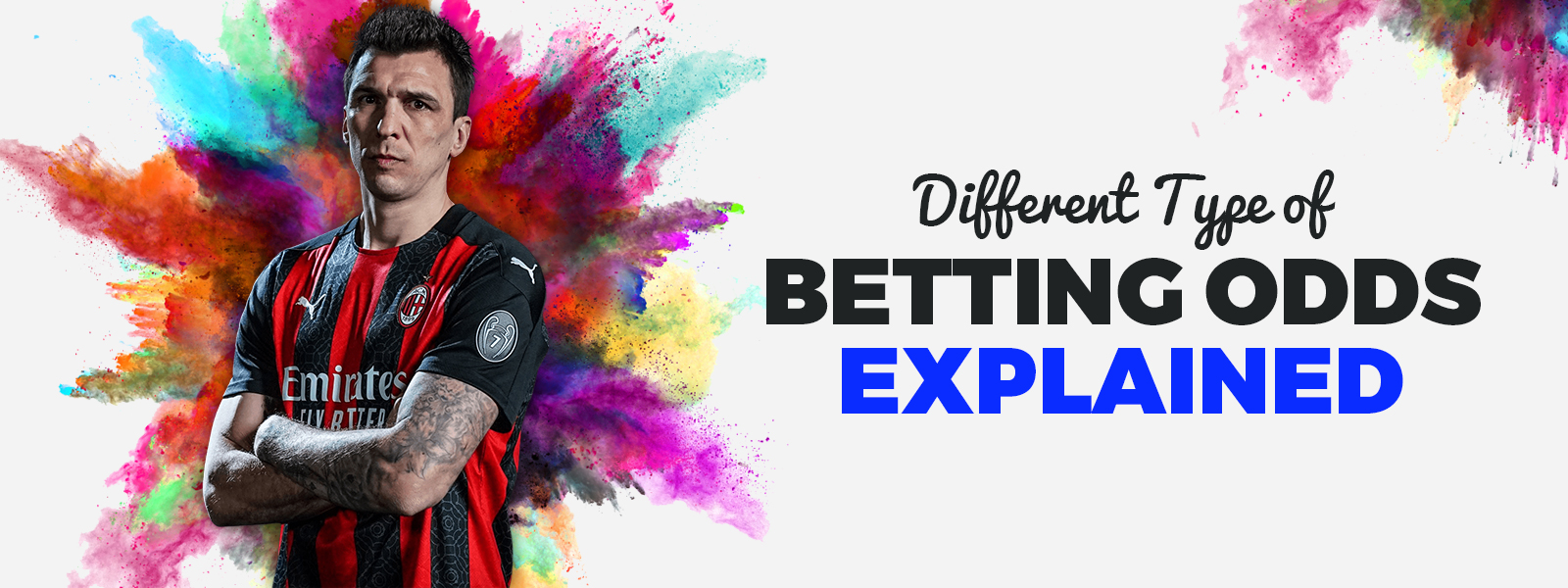Different Type Of Betting Odds Explained
< Back to BlogDifferent Type Of Betting Odds Explained
Date : February 14,2021
Author : Derick Lori Categories :

## Different Type Of Betting Odds

Knowing the concepts used in the betting world is essential for every sports bettor. One of the most important is the betting odds. To handle betting odds properly, it is necessary to know the different formats in which sports bookies display them, allowing you to interpret them correctly. In the sports bookies, we can find three basic betting odds formats. These are the decimal, the fractional, and the American.

### The Decimal Betting Odds Format

The decimal odds format is the most widely used in Europe. They express the gross winnings that we can get by betting on an event. For example, if we bet \$ 20 on a 1.95 odds, our gross winnings will be \$ 20 x 1.95 = \$ 39. To obtain the net profit, we must subtract the amount bet from the gross profit. The net profit in this example would be (\$ 20 x 1.95) - \$ 20 = \$ 19

### The Fractional Betting Odds Format

This way of expressing odds is used mainly in the United Kingdom. The odds have the form of a fraction. The numerator indicates the monetary units you would win, and the denominator the monetary units to wager. For example, a 3/2 odd indicates that we will get \$ 3 for every \$ 2 wagered. An odd equal to 7/1 means that for every dollar wagered, we can win \$ 7, and a 1/3 odd is equivalent to saying that we must risk \$ 3 to win 1.

### The American Odds Format

American odds are presented as whole numbers and are accompanied by a positive sign (+) and a negative sign (-). Positive odds indicate which team is the underdog. Likewise, they demonstrate how much your net winnings will be if you bet \$ 100. For example, if you bet \$ 100 at +120 odds, you would win \$ 120 if you hit your bet. On the other hand, negative odds show the amount of money you have to bet to win \$ 100. These odds are related to the favorite teams.vSuppose we have an odd off -145. In this case, you would have to bet \$ 145 to win \$ 100.

### Converting From a Betting Odds Format To Another

Next, we will see how to go from fractional and American betting odds formats to decimal since the latter is the easiest to use. Changing a fractional odd to decimal format is as simple as dividing the numerator by the fractional odd's denominator and adding one to the result. Let's see it in an example. If we express a 5/2 odds in decimal format, it would be (5/2) + 1 = 3.5 Converting American odds to decimal odds depends on the sign (positive or negative) of the odd:

• From positive American odds to decimal odds:

You must divide the odd value by 100 and add 1 to the result. For example, if the American odd is +120, the decimal odd equals (120/100) + 1 = 2.2.

• From negative American odds to decimal odds:

In this case, we must always divide the number 100 by the odd value without the negative sign. We will add the number 1 to the result. If we have an odd of -110, in the decimal format, it would be equivalent to (100/110) + 1 = 1.91

With this, you already have everything you need to dominate the odds.